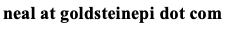# Neal D. Goldstein, PhD, MBI

Jul 1, 2015

### Epi Vignettes: Case-Control Study

A brief synopsis of epidemiologic study design and methods with sample analytic code in R.

In this second installment in the series, I discuss case control sampling and analytic techniques. As before, the intention of this series is to: 1) Briefly describe the study design, 2) Qualitatively talk about the analysis strategy, and 3)Quantitatively demonstrate the analysis and provide sample R code.

• Data description: Assume a binary outcome (case or control), an exposure and several covariates.
• Study design: Case-Control. Sampling in a case-control study is done by the outcome, with the exposure ascertained by retrospective analysis (e.g., asking the participants to recall). While a case-control study can be prospective, those are infrequent; therefore this post will be concerned only with historic exposure assessment. A case, and one or more controls, are sampled from a population with the goal of creating the counter-factual occurrence: the same person had and did not have the outcome. If the controls are as similar as possible to the cases on all other characteristics, in theory the risk factors for disease can be elucidated. Case-control studies are frequently "matched" on one or more covariates to balance the groups on potential confounding factors, in attempt to achieve exchangeability. The matching may be individual, exact matching, or by matching within a range of allowable values, termed frequency matching. Matched designs may require special techniques in the analysis.
• Goal of analysis: Describe the odds of outcome given an exposure. More specifically, we are describing the odds of exposure conditioned on case status (case or control), which mirror the odds of outcome given an exposure. Case-control studies cannot be used to describe the incidence of an outcome, as this is fixed by study design. That is, the researcher controls the ratio of cases to controls.
• Statistical techniques: Logistic regression techniques are appropriate when adjusting or controlling for potential confounding. Otherwise, a standard contingency table can be used to directly calculate the odds of exposure. The measure of association in a logistic regression analysis will be on the log-odds scale, which is then converted to an odds ratio for presentation. The odds ratio is not an intuitive concept; this post will assume the reader is familiar with odds. However, frequently the odds ratio is used synonymously with relative risk, which is incorrect. The odds ratio is a biased estimate of the relative risk, which may approximate the true risk in certain situations (the rare outcome assumption). Consult an epidemiologist if you are uncomfortable with the underlying assumptions of the odds ratio.
• Unconditional logistic regression: This technique is appropriate in an unmatched case-control study, or one where frequency matching was used and multiple controls overlap multiple cases (i.e., a control could be matched to more than one case). The beta estimates for each coefficient in the regression equation are interpreted as the log odds of outcome, given a unit change (or presence of, in the case of categorical variables), and the exponentiated coefficients are interpreted as the corresponding change in odds.
• Conditional logistic regression: This technique is appropriate in a matched study design. It is common to also include the matched variables in the model specification to control for possible residual confounding from the matching process. In essence, the intercept (baseline log odds) is estimated for each matched pair. By failing to specify the matched-pairs, the estimation of parameters would be incorrect (and possibly fail to converge). For each observation (subject) in the dataset, a matched pairs identifier specifies which case (or control) was matched to a given subject. The interpretation of the coefficient estimates is the same as in unconditional logistic regression.

Sample codes in R

Unconditional logistic regression```model = glm(outcome~ exposure+covariates, data=casecontrol, family=binomial(link="logit"))
summary(model) #summary of the model
round(exp(coef(model)),2) #coefficient estimates: odds ratios
round(exp(confint(model)),2) #confidence intervals
```

Conditional logistic regression (package:survival)```model = clogit(outcome~ exposure+covariates+matched_covariates+strata(matched_pairs_identifier), data=casecontrol)
summary(model) #summary of the model
round(exp(coef(model)),2) #coefficient estimates: odds ratios
round(exp(confint(model)),2) #confidence intervals
```

Cite: Goldstein ND. Epi Vignettes: Case-Control Study. Jul 1, 2015. DOI: 10.17918/goldsteinepi.